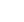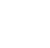# Advanced Statistics for Data Science

## Description

Fundamental concepts in probability, statistics and linear models are primary building blocks for data science work. Learners aspiring to become biostatisticians and data scientists will benefit from the foundational knowledge being offered in this specialization. It will enable the learner to understand the behind-the-scenes mechanism of key modeling tools in data science, like least squares and linear regression. This specialization starts with Mathematical Statistics bootcamps, specifically concepts and methods used in biostatistics applications. These range from probability, distribution, and likelihood concepts to hypothesis testing and case-control sampling. This specialization also linear models for data science, starting from understanding least squares from a linear algebraic and mathematical perspective, to statistical linear models, including multivariate regression using the R programming language. These courses will give learners a firm foundation in the linear algebraic treatment of regression modeling, which will greatly augment applied data scientists' general understanding of regression models. This specialization requires a fair amount of mathematical sophistication. Basic calculus and linear algebra are required to engage in the content.

## Specific details

Category of Education Physical Science

## University

Johns Hopkins University

There are no comments posted here yetinfo@educom.net
 About Terms and ConditionsPrivacy PolicyRankingsAccreditations